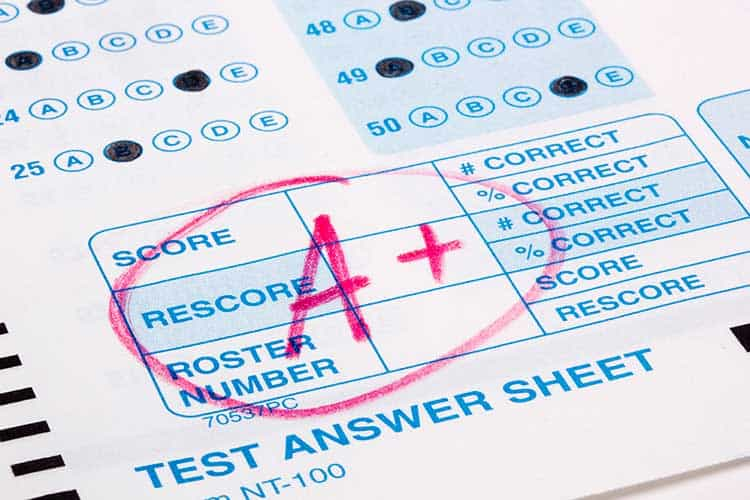PAX® math practice questions, prepared by a dedicated team of exam experts, with detailed answer key, Math shortcuts, tips and tricks, tutorials and multiple choice strategies!

Basic Math

• Basic operations – adding subtracting, multiplying and dividing whole numbers
• Square root
• Prime factors
• Median and mode
• Exponents
• Word problems
• Ratio and proportion
• Fractions, decimals and percent

How to Study for a Math Test

Practice

Basic Math – 10 questions
Basic Math 2 – 10 questions
Word Problems Tutorial with examples
Percent – 18 questions
Metric Conversion – 8 questions
Fractions, Percent and Decimals – 14 questions
Word Problems with Ratio and Proportion

Speed, acceleration and momentum

Algebra

Basic Algebra
Operations with Polynomials
Solving equations with 1 variable

Practice

Operations with Polynomials – 4 questions
Basic Algebra – 7 questions
Linear equations

Basic Statistics

Median, mean and mode
Simple probability

Basic Geometry

Slope
Area, circumference, perimeter
Pythagorean Geometry

Need to brush up on your PAX math? This is the book for you!

PAX® Math practice questions, easy-to-read tutorials explaining everything in plain language, exam tips and tricks, math shortcuts, and multiple choice strategies! Everything you need, compiled by a dedicated team of experts with everything you need all in one place!

PAX®, and the Test of Essential Academic Skills is a registered trademark of the Assessment Technologies Institute, which does not endorse this product.

Here is what the PAX® Math Workbook can do for you:

• Learn then practice your math skills! Practice test questions are the best way to prepare for an exam and this is the book that you need to fully prepare for the PAX math test.
• Practice Tests familiarize you with the exam format and types of questions, giving you more confidence when you take the exam.
• Practice tests are a critical self-assessment tool that reveals your strengths and weaknesses.
• Practice tests allow you to practice your exam time management – a critical exam-writing skill that can easily improve your grade substantially.
• Practice tests reduce Test Anxiety, one of the main reasons for low marks on an exam. Hundreds of questions with detailed solutions and explanations to improve your understand of the basic concepts behind the questions.
• Learn powerful multiple choice strategies designed by exam experts! Includes tips and multiple choice strategies to increase your score you won’t find anywhere else!

Practice Really Does Make Perfect!

The more questions you see, the more likely you are to pass the test. And between our study guide and practice tests, you’ll have over 200 practice questions that cover every category.

Our practice test questions have been developed by our dedicated team of experts. All the material in the study guide, including every practice question, are designed to engage your critical thinking skills needed to pass the test!

Heard it all before?

Maybe you have heard this kind of thing before, and don’t feel you need it. Maybe you are not sure if you are going to buy this book.

Remember though, it only a few percentage points divide the PASS from the FAIL students!

Even if our test tips increase your score by a few percentage points, isn’t that worth it?Reviews: 5 Stars

• Hundreds of Practice Questions
• Multiple Choice Tips and Strategies from Exam Experts
• Self-Assessments to Identify your Strength and Weaknesses
• How to make a Study Plan and Study Schedule
• Practice Test Questions for Basic Math, Advanced Math, Algebra, Word Problems, Statistics, Geometry and more! .
• How to Study for a Math Test
• And a Lot More!PAX Math exercises, tutorials and multiple choice strategies

Over 200 PAX Math practice questions, plus test tips, how to study math, multiple choice strategies and more!

Format:
Condition: New
Availability: In Stock

Written by,

Date Published: Saturday, April 8th, 2017
Date Modified: Friday, August 20th, 2021

Good!Good!

Reviewed in the United States on May 25, 2020

It’s good practice. Something you might need to refresh what you learned in high school. For me it’s been 5 years since I was in high school and need a refresher.

Contents

Getting Started
The PAX Study Plan 9
Making a Study Schedule 12

Basic Operations
Practice Questions 17

Estimation
Practice Questions 27

Graphs and Tables
Practice Questions 34

Word Problems
How to Solve Word Problems 41
Types of Word Problems 44

Fractions, Decimals and Percent
Fraction Tips and Shortcuts 75
Fractions Decimal & Percent 75
Decimal Tips and Shortcuts 81
Percent Tips, Tricks and Shortcuts 82
Practice Questions 86

Metric Conversion
Practice Questions 102

Basic Algebra
Solving One-Variable Equations 107
Simplifying Polynomials 108
Factoring Polynomials 109
Basic Algebra Practice 113

Geometry
Cartesian Plane, Grid and Plane 136
Pythagorean Geometry 142
Geometry Practice Questions 149

Speed, Force and Momentum
Practice Questions 182

Mode, Mean and Median
Practice Questions 190

Basic Math Multiple Choice

How to Study for a Math Test

How to Prepare for a Test

How to Take a Test